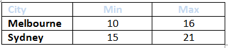# Developer Checklist

The following checklists are intended to guide developers on the implementation of success criterion for tables. The checklists should be used during the development phase to ensure WCAG 2.0 compliance of all tables on the site and should form part of the unit testing phase undergone by developers when updating or creating new content or functionality.

## Level A – Data tables

### Data tables are used correctly

#### TBL_A1i

Details
Empty tables are not used
Correct example(s)
``````<table summary="Minimum and maximum temperatures for Australian state capital cities" Caption="Temperature comparison by city">
<tr>
<th>City</th>
<th>Min</th>
<th>Max</th>
</tr>
<tr>
<td>Melbourne</td>
<td>10</td>
<td>16</td>
</tr>
<tr>
<td>Sydney</td>
<td>15</td>
<td>21</td>
</tr>
</table>``````
Incorrect example(s)
``<table> </table>``

#### TBL_A1ii

Details
Excessive nesting of tables is avoided
Correct example(s)
``````<table summary="Minimum and maximum day and nightly temperatures for Australian state capital cities" Caption="Day and night temperature comparison by city">
<tr>
<th id="city">City rowspan="2"</th>
<th id="min">Minimum temperature</th>
<th id="max">Maximum temperature</th>
</tr>
<tr>
</tr>
<tr>
</tr>
<tr>
</tr>
</table>``````
Incorrect example(s)
``````<table summary="Minimum and maximum day and nightly temperatures for Australian state capital cities"Caption="Day and night temperature comparison by city">
<tr>
<th>Minimum temperature</th>
<th>Maximum temperature</th>
</tr>
<tr>
<td>
<table>
<tr>
<th>City</th>
<th>Day</th>
<th>Night</th>
</tr>
<tr>
<td>Melbourne</td>
<td>10</td>
<td>5</td>
</tr>
<tr>
<td>Sydney</td>
<td>16</td>
<td>10</td>
</tr>
</table>
</td>
<td>
<table>
<tr>
<th>City</th>
<th>Day</th>
<th>Night</th>
</tr>
<tr>
<td>Melbourne</td>
<td>21</td>
<td>15</td>
</tr>
</tr>
<tr>
<td>Sydney</td>
<td>25</td>
<td>10</td>
</tr>
</table>
</td>
</tr>
</table>``````

### Data tables are coded correctly

#### TBL_A2i

Details
Simple data tables are coded with header (`TH`) cells
Correct example(s)
``<tr><th>Location</th><th>Min</th><th>Max</th></tr>``
Incorrect example(s)
``<tr><td>Location</td><td>Min</td><td>Max</td></tr>``

#### TBL_A2ii

Details
Data table header (`TH`) cells contain content i.e. are not empty
Correct example(s)
``<tr><th>Location</th><th>Min</th><th>Max</th></tr>``
Incorrect example(s)
``<tr><th> </th><th>Min</th><th>Max</th></tr>``

#### TBL_A2iii

Details
Data table header (`TH`) cells are descriptive
Correct example(s)
``<tr><th>City</th><th>Minimum temperature</th><th>Maximum temperature</th></tr>``
Incorrect example(s)
``<tr><th>Loc</th><th>Min</th><th>Max</th></tr>``

#### TBL_A3i: Data tables have a `SUMMARY`

Details
Data tables are coded with a `SUMMARY` attribute
Correct example(s)
``<table summary="Minimum and maximum temperatures for Australian state capital cities">``
Incorrect example(s)
``<table>``

#### TBL_A3ii

Details
The data table `SUMMARY` attribute is descriptive i.e. the `SUMMARY` attribute should summarise the content of the data table
Correct example(s)
``<table summary="Minimum and maximum temperatures for Australian state capital cities for today">``
Incorrect example(s)
`SUMMARY` is not descriptive
``<table summary="City temperatures"``
`SUMMARY` is empty
``<table summary=" "> ``

### Data tables have a `CAPTION`

#### TBL_A4i

Details
Data tables are coded with a `CAPTION` element
Correct example(s)
``<caption>City temperatures</caption>``
Incorrect example(s)
Missing `<caption>`

#### TBL_A4ii

Details
The data table `CAPTION` is descriptive i.e. the `CAPTION` element should name the data table
Correct example(s)
``<caption>Temperature comparison by city</caption>``
Incorrect example(s)
`CAPTION` is not descriptive
``<caption>Temperatures</caption>``
`CAPTION` is empty
``<caption></caption>``

#### TBL_A4iii

Details
The data table `CAPTION` is different to the `SUMMARY`
Correct example(s)
``````<table summary="Minimum and maximum temperatures for Australian state capital cities">
<caption>Temperature comparison by city</caption>``````
Incorrect example(s)
``````<table summary="Temperature comparison by city">
<caption>Temperature comparison by city</caption>``````

#### TBL_A5

Details
All rows in the data table contain information i.e. there should be no empty rows
Correct example(s)
``````<table summary="Minimum and maximum temperatures for Australian state capital cities">
<Caption>Temperature comparison by city></caption>
<tr>
<th>City</th>
<th>Minimum temperature</th>
<th>Maximum temperature</th>
</tr>
<tr>
<td>Melbourne</td>
<td>10</td>
<td>16</td>
</tr>
<tr>
<td>Sydney</td>
<td>15</td>
<td>21</td>
</tr>
</table>``````
Incorrect example(s)
``````<table summary="Minimum and maximum temperatures for Australian state capital cities">
<caption>Temperature comparison by city></caption>
<tr>
<th>City</th>
<th>Minimum temperature</th>
<th>Maximum temperature</th>
</tr>
<tr>
<td>Melbourne</td>
<td>10</td>
<td>16</td>
</tr>
<tr>
<td></td>
<td></td>
<td></td>
</tr>
<tr>
<td>Sydney</td>
<td>15</td>
<td>21</td>
</tr>
</table>``````

### Complex tables are coded correctly

#### TBL_A6

Details
Complex data tables are coded with `TH ID` and `TD HEADERS`.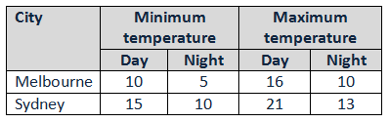Correct example(s)
``````<tr>
<th id="city" rowspan="2">City</th>
<th id="min">Minimum temperature</th>
<th id="max">Maximum temperature</th>
</tr>
<tr>
</tr>
<tr>
</tr>
<tr>
</tr>
</table>``````
Incorrect example(s)
``````<tr>
<th rowspan="2">City</th>
<th>Minimum temperature</th>
<th>Maximum temperature</th>
</tr>
<tr>
<th>Day</th>
<th>Night</th>
<th>Day</th>
<th>Night</th>
</tr>
<tr>
<th>Melbourne</th>
<td>10</td>
<td>5</td>
<td>16</td>
<td>10</td>
</tr>
<tr>
<th>Sydney</th>
<td>15</td>
<td>10</td>
<td>21</td>
<td>13</td>
</tr>
</table>``````

### Complex tables can be understood

#### TBL_A7

Details
Complex data tables are supported by additional explanatory information within the page content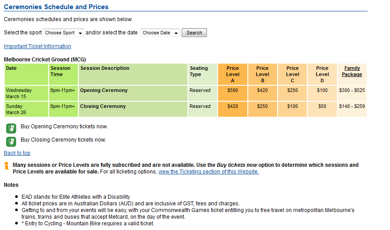Correct example(s)
``<table class="scheduleprice" summary="This table is used to assist in the layout to display sport schedules and prices.">…``
Incorrect example(s)
`` <table class="scheduleprice" summary="This table is used to display sport schedules and prices.">…``

### Table content is distinguishable

#### TBL_A8

Details
Supplementary formatting or information is provided in addition to colour and/or shape and/or shading.
Correct example(s)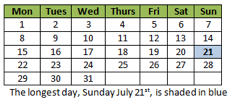Incorrect example(s)## Level A – Layout tables

### Layout tables are used appropriately

#### TBL_A9i

Details
Tables are not used in preference to MathML to present equations
Correct example(s)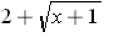is coded:
``````[itex]
<mrow>
<mn>2</mn>
<mo>+</mo>
<msqrt>
<mi>x</mi>
<mo>+</mo>
<mn>1</mn>
</msqrt>
</mrow>
[/itex]``````
Incorrect example(s)
 2 + / x+1
``````<table>
<tr>
<td>2</td>
<td>+</td>
<td>/ x+1</td>
</tr>
</table>``````

#### TBL_A9ii

Details
Layout tables must have more than one cell
Correct example(s)
``<p>Melbourne is the State capital of Victoria</p>``
Incorrect example(s)
``````<table>
<tr>
<td>Melbourne is the State capital of Victoria</td>
<tr>
</table>``````

### Layout tables are coded correctly

#### TBL_A10i

Details
Layout tables are not coded with a `SUMMARY` attribute
Correct example(s)
``````<table>
<tr>
<td>Melbourne</td>
<td>State capital of Victoria</td>
</tr>
<tr>
<td>Sydney</td>
<td>State capital of New South Wales</td>
</tr>
</table>``````
Incorrect example(s)
``````<table summary="State capitals">
<tr>
<td>Melbourne</td>
<td>State capital of Victoria</td>
</tr>
<tr>
<td>Sydney</td>
<td>State capital of New South Wales</td>
</tr>
</table>``````

#### TBL_A10ii

Details
Layout tables are not coded with a `CAPTION` element
Correct example(s)
``````<table>
<tr>
<td>Melbourne</td>
<td>State capital of Victoria</td>
</tr>
<tr>
<td>Sydney</td>
<td>State capital of New South Wales</td>
</tr>
</table>``````
Incorrect example(s)
``````<table>
<caption>State capitals</caption>
<tr>
<td>Melbourne</td>
<td>State capital of Victoria</td>
</tr>
<tr>
<td>Sydney</td>
<td>State capital of New South Wales</td>
</tr>
</table>``````

#### TBL_A10iii

Details
Layout tables do not have table headers: `TH`
Correct example(s)
``````<table>
<tr>
<td>Melbourne</td>
<td>State capital of Victoria</td>
</tr>
<tr>
<td>Sydney</td>
<td>State capital of New South Wales</td>
</tr>
</table>``````
Incorrect example(s)
``````<table>
<tr>
<th>Melbourne</th>
<td>State capital of Victoria</td>
</tr>
<tr>
<th>Sydney</th>
<td>State capital of New South Wales</td>
</tr>
</table>``````

#### TBL_A11

Details
Layout tables used to present textual content are in meaningful sequence i.e. make sense when read cell to cell from left to right
Correct example(s)
``````<table>
<tr>
<td>Melbourne</td>
<td>State capital of Victoria</td>
</tr>
<tr>
<td>Sydney</td>
<td>State capital of New South Wales</td>
</tr>
</table>``````
Incorrect example(s)
``````<table>
<tr>
<td>Melbourne</td>
<td>Sydney</td>
</tr>
<tr>
<td>State capital of Victoria</td>
<td>State capital of New South Wales</td>
</tr>
</table>``````

## Level AA

### Colour Contrast

#### TBL_AA1: Colour contrast

Details
Colour contrast within table content is sufficient
Correct example(s)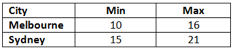Incorrect example(s)